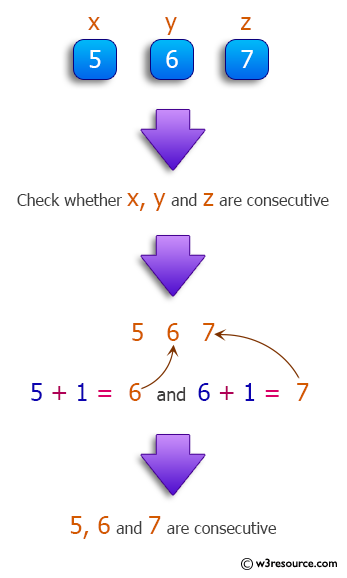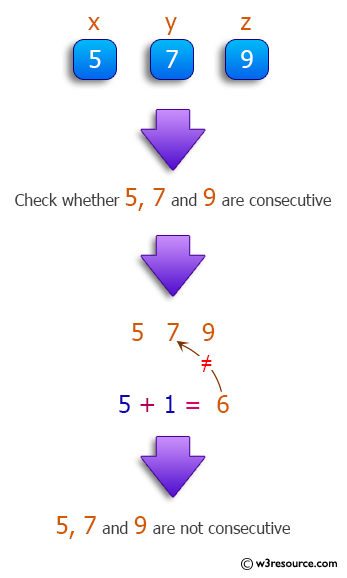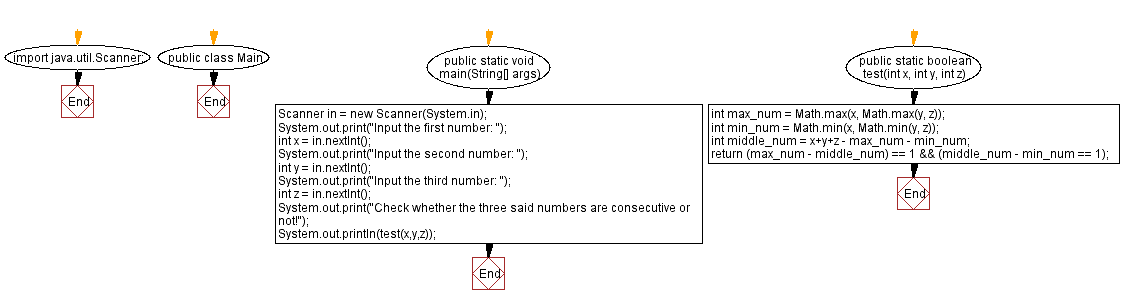﻿ Java: Three integers and check whether they are consecutive# Java Method Exercises: Three integers and check whether they are consecutive

## Java Method: Exercise-18 with Solution

From Wikipedia-
Consecutive numbers are numbers that follow each other in order. They have a difference of 1 between every two numbers. In a set of consecutive numbers, the mean and the median are equal.
If n is a number, then the next numbers will be n+1 and n+2.

Write a Java method that accept three integers and check whether they are consecutive are not. Return true or false.

Pictorial Presentation:Examples:
Consecutive numbers that follow each other in order:
1, 2, 3, 4, 5
-3, −2, −1, 0, 1, 2, 3, 4
6, 7, 8, 9, 10, 11, 12, 13

Sample Solution:

Java Code:

``````import java.util.Scanner;
public class Main {
public static void main(String[] args)
{
Scanner in = new Scanner(System.in);
System.out.print("Input the first number: ");
int x = in.nextInt();
System.out.print("Input the second number: ");
int y = in.nextInt();
System.out.print("Input the third number: ");
int z = in.nextInt();
System.out.print("Check whether the three said numbers are consecutive or not!");
System.out.println(test(x,y,z));
}

public static boolean test(int x, int y, int z){
int max_num = Math.max(x, Math.max(y, z));
int min_num = Math.min(x, Math.min(y, z));
int middle_num = x+y+z - max_num - min_num;
return (max_num - middle_num) == 1 && (middle_num - min_num == 1);
}
}
```
```

Sample Output:

```Input the first number:  15
Input the second number:  16
Input the third number:  17
Check whether the three said numbers are consecutive or not!true
```

Flowchart :Java Code Editor:

Previous Java Exercise: In an integer, count the number of digits with value 2.
Next Java Exercise: Accept three integers and return the middle one.

What is the difficulty level of this exercise?

Test your Programming skills with w3resource's quiz.

﻿